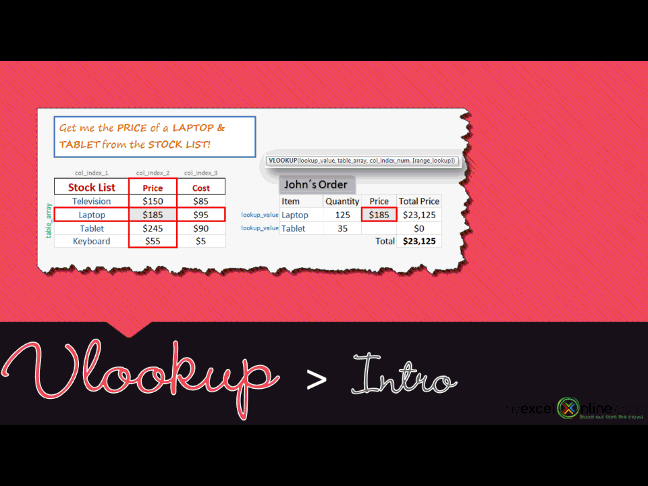## Want to know how to use the VLOOKUP function?

*** Watch our video and step by step guide below with free downloadable Excel workbook to practice ***

Watch on YouTube and give it a thumbs up 👍What does it do?

Searches for a value in the first column of a table array and returns a value in the same row from another column (to the right) in the table array.

Formula breakdown:

=VLOOKUP(lookup_value, table_array, col_index_num, [range_lookup])

What it means:

=VLOOKUP(this value, in this list, and get me value in this column, Exact Match/FALSE/0])

Excel`s VLOOKUP function is arguably the most used function in Excel but can also be the most tricky one to understand.  I will show you a VLOOKUP example and in a few steps you will be able to extract values from a table and use them to do your custom reports and analysis.

You will be using VLOOKUP with confidence after this tutorial!

STEP 1: We need to enter the VLOOKUP function in a blank cell:

## =VLOOKUP(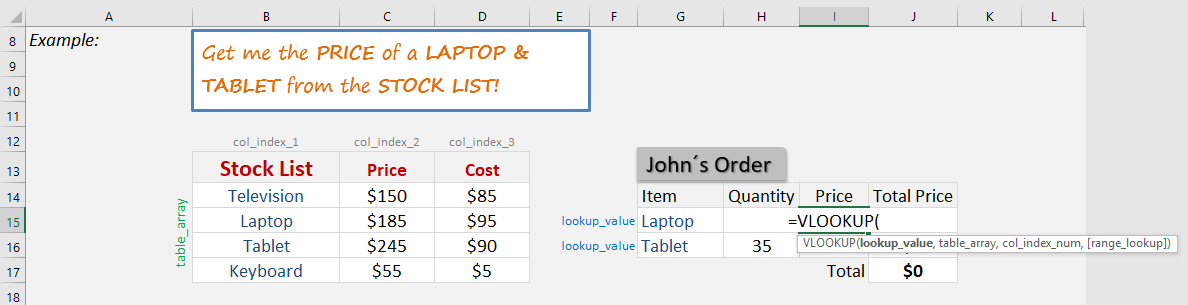STEP 2: The VLOOKUP arguments:

## Lookup_value

What is the value that you want to look for?

In our first example, it will be Laptop, so select the Item name

## =VLOOKUP(G15,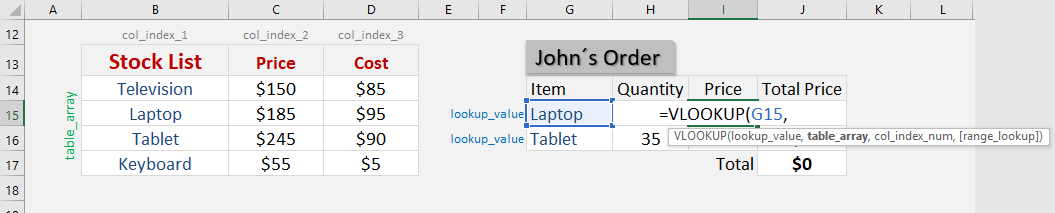## Table_array

What is the table or range that contains your data?

Make sure to select the stock list table so that our VLOOKUP formula will search here

## =VLOOKUP(G15, B14:D17,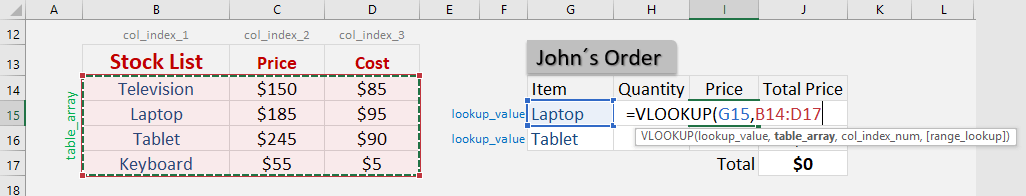Ensure that you press F4 so that you can lock the table range.

## =VLOOKUP(G15, \$B\$14:\$D\$17,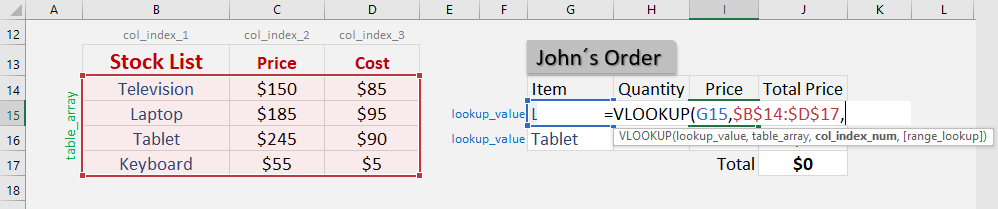## Col_index_num

What is the column that you want to retrieve the value from?

Since we want to get the price, our price is on the 2nd column of our source data

## =VLOOKUP(G15, \$B\$14:\$D\$17, 2,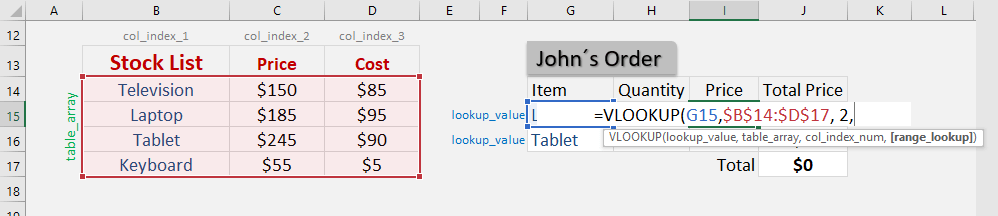## Range_lookup

What kind of matching do you need?

We want an exact match of the Laptop text so make sure FALSE is selected.

## =VLOOKUP(G15, \$B\$14:\$D\$17, 2, FALSE)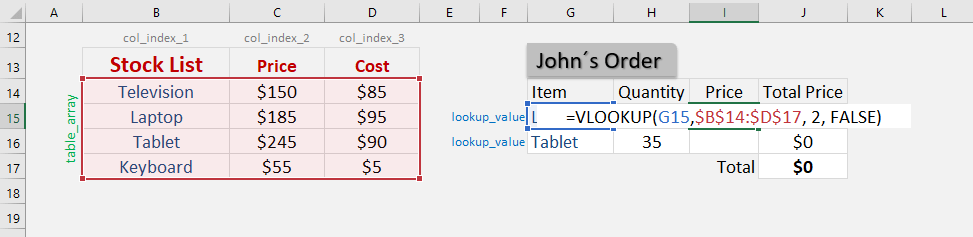Apply the same formula to the rest of the cells by dragging the lower right corner downwards.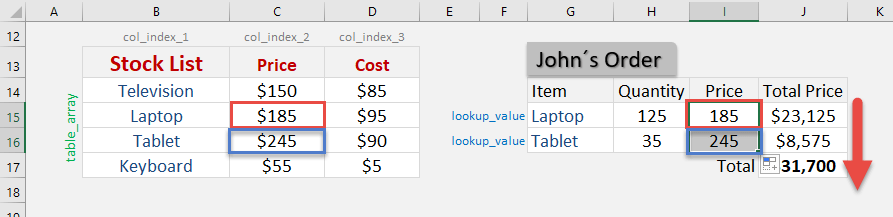You now have all of the results!

How to Use the VLOOKUP Formula in Excel